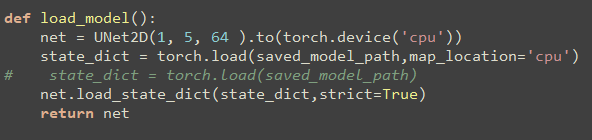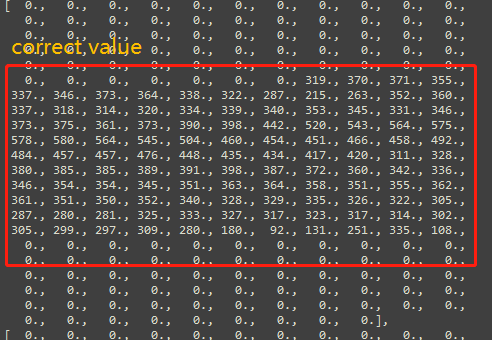# Having problems in segmenting image when using libtorch

I build a U-Net for segmenting medical images in pytorch 1.3. There’s no problems in training and testing stages when using pytorch. Here is my codes for converting ‘model.pth’ to ‘model.pt’, and ‘model.pt’ will apply in libtorch for testing with C++ later.and here is my test code for evaluate my model.

everything goes fine in pytorch, and i can get the correct result in python code.
However, when i code in C++ with using libtorch, i can’t get the same result in python code. Here is my C++ code for testing.

I check every stage output in libtorch and pytorch. I find that in pytorch, after ‘predict = net(test_data)’, the tensor is:

But in C++, there’re werid values appear after ‘auto result = module.forward(inputs).toTensor();’, these values change into NaN, here is the result output:

so i go back to check my image input, i also find in pytorch, data input is:there is another value such as 255.0/128.0 in tensor. But in C++, there are manys of huge values:

therefore i get a full black image output in C++ while a nice result in python.I wonder how to do segmentation in libtorch with my case. Thanks!

I had similar issues before. Turns out I was passing CPU data into loaded model’s forward function. Make sure your inputs are in CUDA.

Edit: Nevermind I just realized you also defined your network in CPU. However most of my nan output cases occured because I gave the wrong input type. It would be good to check the data type and which device it is in both Python and C++.

Thanks for your attention! I defined my network in CPU which is in both Python and C++.`torch::jit::script::Module module = torch::jit::load(model_path,torch::kCPU);`

I still get nan output. So i agree that maybe input data type is wrong:

``````    test_data = torch.from_numpy(test_data)
test_data = torch.tensor(test_data,dtype=torch.float32)
``````
``````	torch::Tensor tImage = torch::from_blob(src1.data, { 240,240,1 }, torch::kF32);
tImage = tImage.permute({ 2,0,1 });
tImage = tImage.div(255);
tImage = tImage.unsqueeze(0);
``````

Annoyingly, nan output still occures.I may find where cause these problems.
In pytorch, after transforming numpy to tensor, the image value doesn’t change expect int16 to float32:

``````    test_data = np.asarray(nda)        # int16
test_data = torch.from_numpy(test_data)
test_data = torch.tensor(test_data,dtype=torch.float32) #to float32
test_data=torch.unsqueeze(test_data,0)
test_data=torch.unsqueeze(test_data,1)
``````But in libtorch, i implement the same progress with code:

``````        torch::Tensor tImage = torch::from_blob(src.data, { 240,240,1 }, torch::kFloat32);
tImage = tImage.permute({ 2,0,1 });
tImage = tImage.div(255);
tImage = tImage.unsqueeze(0);
cout << "prob:" << tImage.data() << endl;
vector<torch::jit::IValue> inputs;  //def an input

inputs.push_back(torch::ones({ 1,1,240,240 }).toType(torch::kFloat32));
``````

and i get different values:

i don’t exactly know whether is the key point or not. i need help.

Edit: those C++ output vaules are the result of ‘torch.div(255)’. i think it is not the key point.